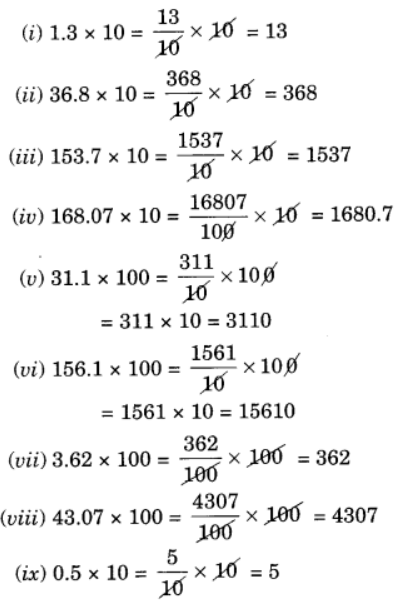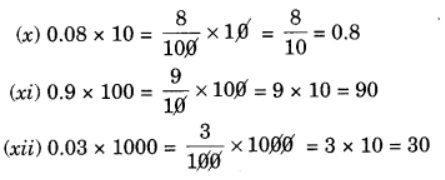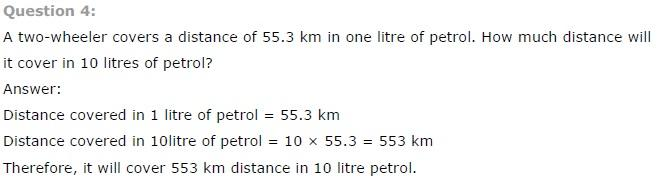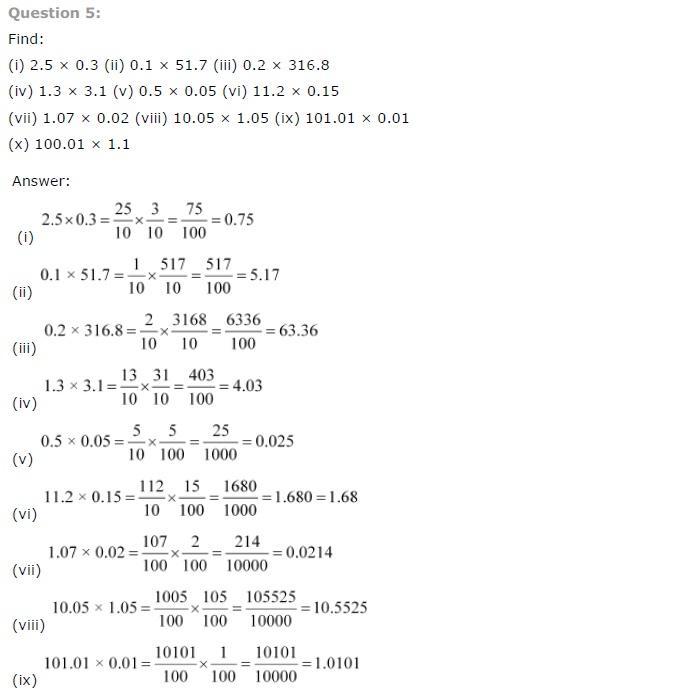# Class 7 Maths Fractions and Decimals Exercise 2.6

## NCERT Solutions class 7 Maths Chapter 2 Fractions and Decimal

### Fractions and Decimal Exercise-2.6

NCERT Solutions Class-7 Maths chapter 2 Fractions and Decimals Exercise-2.6 is prepared by academic team of Physics Wallah all the questions of NCERT text book are solved step by step with proper and detail solutions explaining each and every questions. For More and additional questions of CBSE class 7 maths you can go to class 7 maths sections. NCERT class 7 Maths Solutions is the best way to enhanced your mathematics skill. And Physics Wallah practice worksheet & question bank will help you a lot .

### Solutions of Chapter Fractions and Decimals Exercise-2.6

Question 1:

Find:

1. 0.2 × 6
2. 8 × 4.6
3. 2.71 × 5
4. 20.1 × 4
5. 0.05 × 7
6. 211.02 × 4
7. 2 × 0.86

Solution:

(i) 0.2 × 6

∵ 2 × 6 = 12 and we have 1 digit right to the decimal point in 0.2.
Thus 0.2 × 6 = 1.2

(ii) 8 × 4.6
∵ 8 × 46 = 368 and there is one digit right to the decimal point in 4.6.
Thus 8 × 4.6 = 36.8

(iii) 2.71 × 5
∵ 271 × 5 = 1355 and there are two digits right to the decimal point in 2.71.
Thus 2.71 × 5 = 13.55

(iv) 20.1 × 4
∵ 201 × 4 = 804 and there is one digit right to the decimal point in 20.1.
∵ 20.1 × 4 = 80.4

(v) 0.05 × 7
∵ 5 × 7 = 35 and there are 2 digits right to the decimal point in 0.05.
Thus 0.05 × 7 = 0.35

(vi) 211.02 × 4
∵ 21102 × 4 = 84408 and there are 2 digits right to the decimal point in 211.02.
Thus 211.02 × 4 = 844.08

(vii) 2 × 0.86
∵ 2 × 86 = 172 and there are 2 digits right to the decimal point in 0.86.
Thus 2 × 0.86 = 1.72

Question 2:

Find the area of rectangle whose length is 5.7 cm and breadth is 3 cm.

Length = 5.7 cm
Area of rectangle = length × breadth
= 5.7 × 3 = 17.1 cm2
Hence, the required area =17.1 cm2

Question 3:

Find:

1. 1.3 × 10
2. 36.8 × 10
3. 153.7 × 10
4. 168.07 × 10
5. 31.1 × 100
6. 156.1 × 100
7. 3.62 × 100
8. 43.07 × 100
9. 0.5 × 10
10. 0.08 × 10
11. 0.9 × 100
12. 0.03 × 1000